This tutorial is for building an ionizing radiation detector with Arduino!

BeginnerFull instructions provided1.5 hours499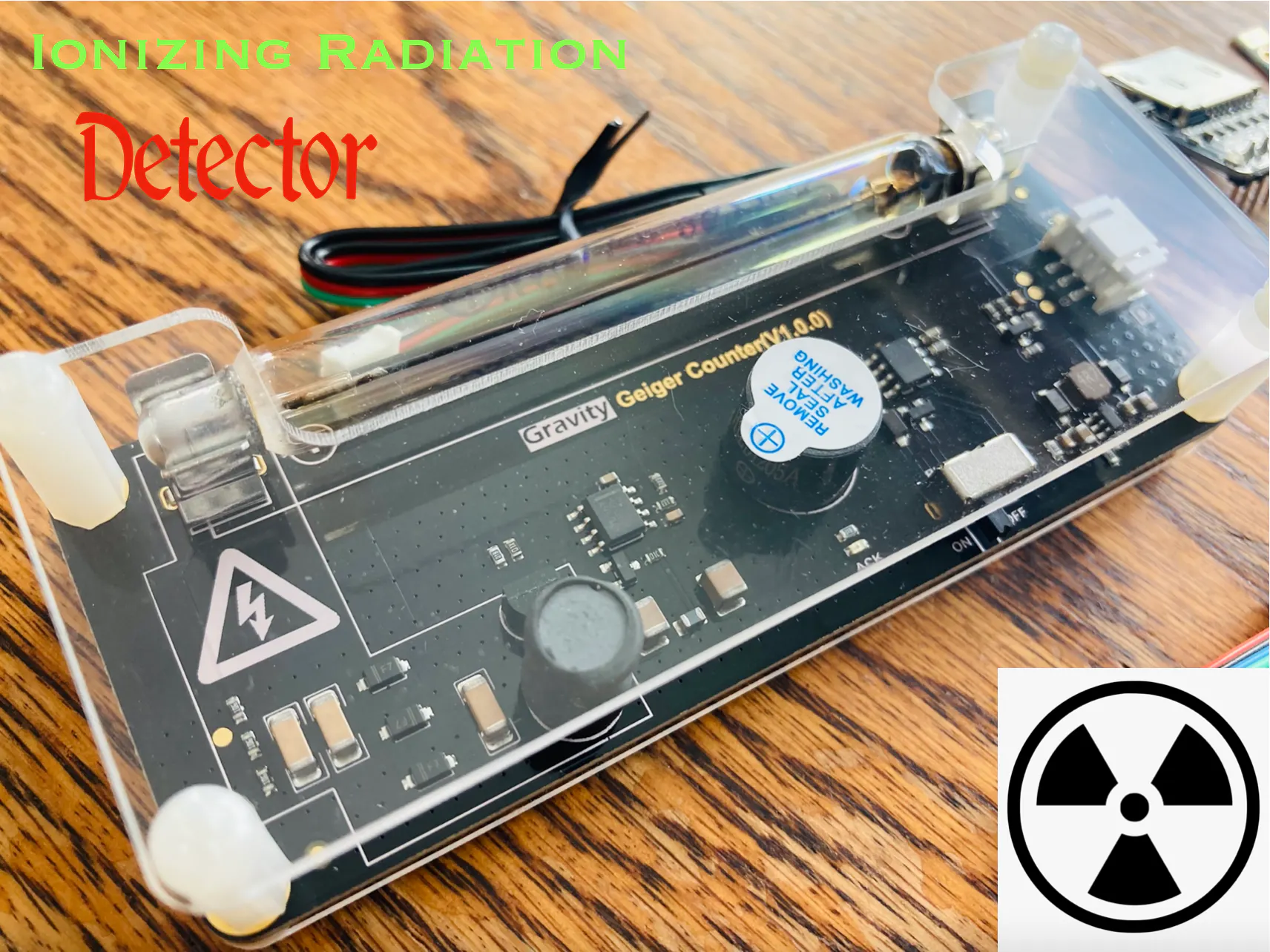## Things used in this project

### Hardware components

 DFRobot Beetle CM-32U4
×1
 DFRobot MicroSD Card Breakout
×1
 DFRobot MicroSD Card
×1
 DFRobot GPS Module
×1
 DFRobot Vibration Motor Module
×1
 DFRobot I2C 16x2 LCD
×1
 DFRobot Geiger Counter Module
×1
 DFRobot DC-DC Converter Only needed if you power this device from a 12v source.
×1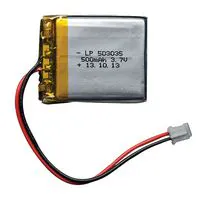Rechargeable Battery, 3.7 V
×1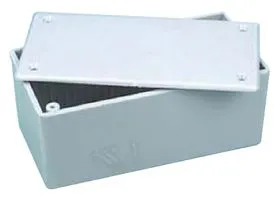Plastic Enclosure, Project Box
×1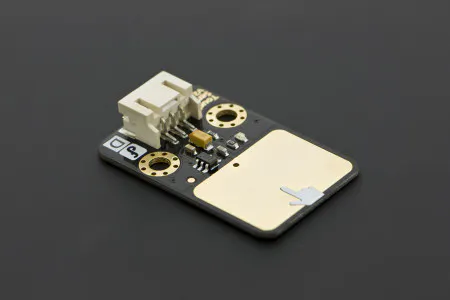DFRobot Gravity: Digital Capacitive Touch Sensor For Arduino
×1

### Hand tools and fabrication machines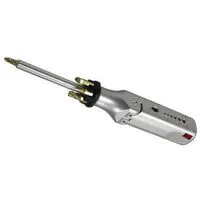Multitool, Screwdriver

## Schematics

### Schematic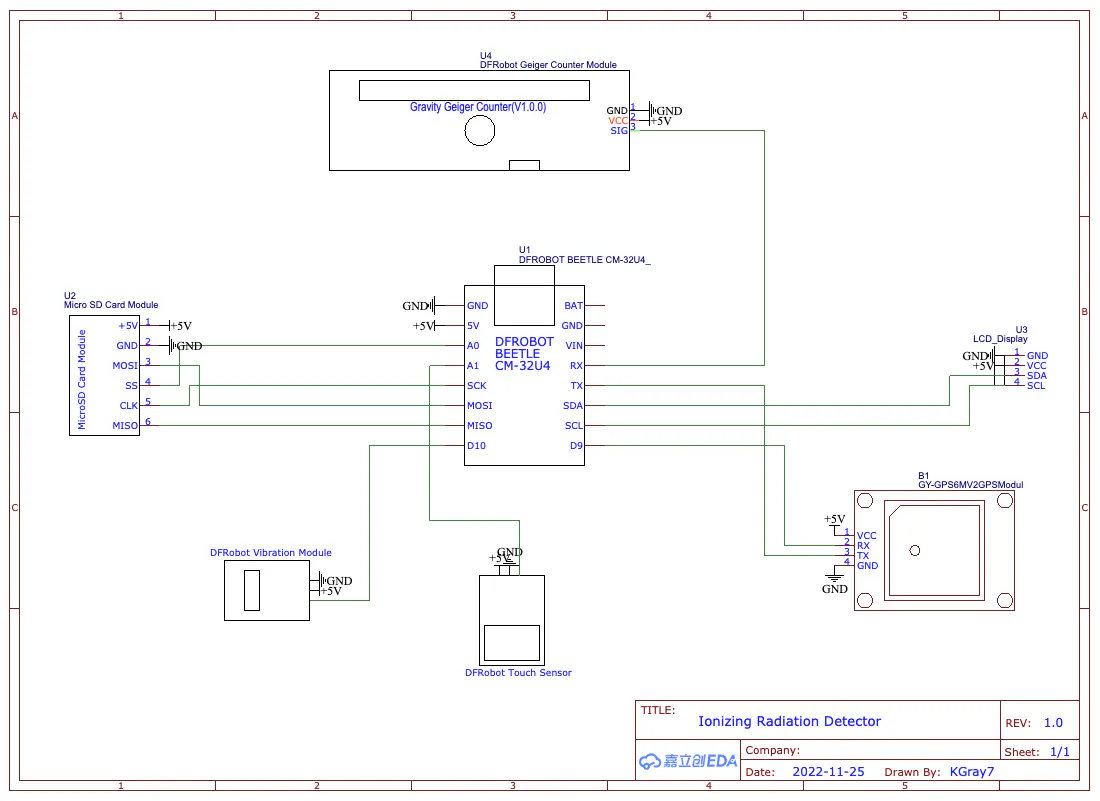## Code

### geiger.ino

C/C++
```#include <DFRobot_RGBLCD1602.h>
#include <TinyGPS++.h>
#include <SoftwareSerial.h>
#include <SD.h>

#define geiger_pin 0
#define button A1
#define vibration 11
#define SDss A0

static const int RXPin = 9, TXPin = 1;//D1/D9
static const uint32_t GPSBaud = 9600;

const byte motor1Char = {
0b00110,
0b00110,
0b00100,
0b01110,
0b01110,
0b01110,
0b01110,
0b01110
};

const byte motor2Char = {
0b01100,
0b01100,
0b00100,
0b01110,
0b01110,
0b01110,
0b01110,
0b01110
};

const byte fullChar = {
0b11111,
0b11111,
0b11111,
0b11111,
0b11111,
0b11111,
0b11111,
0b11111
};

boolean vib = true;
boolean seconds = true;

int i = 0;
const int n = 0;
volatile long buf[n];
float timebetweenpulses = 0;
int pos = 0;

long lastmillis = 0;
long currentmillis = 0;
int looptimerlength = 30000;//30 sec

float lastUSVH = 0;
float CPM = 0;
float USVH = 0;

int mode = 1;

int timer1 = 0;
int count = 0;
int count2 = 0;

#define arrSize(X) sizeof(X) / sizeof(X)

float milli[] =            { 2000,     1000,       900,      800,        700,        600,      500,       400,          300,           100,       50     };
float values[] =           {   0,        1,         2,        5,          10,         40,      100,       250,          400,          1000,      10000   };
const char* equivalent[] = {"Normal", "Normal", "Airport", "Dental", "Norm(1day)", "Flight", "X-Ray", "NPP(1year)", "Mammogram",  "Gov Limit", "CT Scan" };

File myFile;

DFRobot_RGBLCD1602 lcd(/*lcdCols*/16,/*lcdRows*/2);
TinyGPSPlus gps;
SoftwareSerial ss(RXPin, TXPin);

void setup() {
pinMode(geiger_pin, INPUT);
pinMode(vibration, OUTPUT);
pinMode(SDss, OUTPUT);
pinMode(button, INPUT);
digitalWrite(vibration, LOW);

ss.begin(GPSBaud);

lcd.init();
lcd.customSymbol(1,motor1Char);
lcd.customSymbol(2,motor2Char);
lcd.customSymbol(4,fullChar);

lcd.setCursor(4,0);
lcd.print(F("Geiger"));
lcd.setCursor(3,1);
lcd.print(F("Counter"));
lcd.setRGB(0,255,0);
delay(3000);
for (int p=0;p<2;p++){
for (int p=255;p>0;p--) {
lcd.setRGB(0, p, 0);
delay(3);
}
for (int p=0;p<255;p++) {
lcd.setRGB(0, p, 0);
delay(3);
}
}
for (int i=0;i<15;i++){
lcd.scrollDisplayRight();
delay(100);
}

if (!SD.begin(SDss)) {
lcd.clear();
lcd.setRGB(255, 0, 0);
lcd.setCursor(1,0);
lcd.print(F("SD Init Failed"));
delay(5000);
lcd.clear();
}

if (myFile){
myFile.println(F("ID,Name,Date,Time,Latitude,Longitude,CPM,uSvh"));
myFile.close();
}
else {
}
if (myFile){
myFile.println(F("ID,Name,Date,Time,Latitude,Longitude,TimeBPulses"));
myFile.close();
}
else {
}

attachInterrupt(digitalPinToInterrupt(geiger_pin), isrcount, FALLING);

}

void loop() {
while (ss.available() > 0){
}

if (gps.location.isUpdated()){
Serial.print("Latitude: ");
Serial.println(gps.location.lat(),6);
Serial.print("Longitude: ");
Serial.println(gps.location.lng(),6);
}

currentmillis = millis();
if ((currentmillis - lastmillis) >= looptimerlength){
CPM = pos*(60000/looptimerlength);
USVH = (pos*(60000/looptimerlength))/153.80;
Serial.print("CPM: ");
Serial.println(CPM);
Serial.print("uSv/h: ");
Serial.println(USVH);
lastUSVH = USVH;
pos=0;
lastmillis = millis();
if (mode == 3){
lcdDisplayData(3);
if (vib){
if (USVH > 2){
digitalWrite(vibration, HIGH);
delay(300);
digitalWrite(vibration, LOW);
}
}
}
saveToFile(1);
}

if (mode == 1){
lcdDisplayData(1);
}
else if (mode == 2){
lcdDisplayData(2);
}
}

int taps = 0;
redo:

taps++;
if (taps >= 6){
taps = 1;
}
Serial.print("Taps: ");
Serial.println(taps);

do {
timer1++;
delay(1);
if (timer1 > 2000){
timer1 = 0;
goto exi;
}

timer1 = 0;
goto redo;
}
exi:
Serial.print("Completed Taps: ");
Serial.println(taps);

lcd.clear();

if (taps == 1){
lcd.setRGB(0, 255, 0);
lcd.setCursor(0,0);
lcd.print(F("Standard Mode"));
delay(3000);
mode = 1;
}
else if (taps == 2){
lcd.setRGB(0, 255, 255);
lcd.setCursor(3,0);
lcd.print(F("Bar Mode"));
delay(3000);
mode = 2;
}
else if (taps == 3){
lcd.setRGB(0, 0, 255);
lcd.setCursor(0,0);
lcd.print(F("Equivalents Mode"));
delay(3000);
lcd.clear();
lcd.setRGB(0, 255, 0);
lcd.setCursor(0,0);
lcd.print(F("Acquiring Data"));
mode = 3;
}
else if (taps == 4){
vib = !vib;
lcd.setRGB(255, 0, 255);
lcd.setCursor(3,0);
lcd.print(F("Vibration"));
lcd.setCursor(4,1);
lcd.print(vib > 0 ? "On" : "Off");
for (int i=0;i<10;i++){
lcd.setCursor(14,1);
lcd.write(1);
delay(300);
lcd.setCursor(14,1);
lcd.write(2);
delay(300);
}
mode = 1;
}
else if (taps == 5){
seconds = !seconds;
lcd.setRGB(255, 0, 255);
lcd.setCursor(3,0);
lcd.print(F("Seconds"));
lcd.setCursor(4,1);
lcd.print(seconds > 0 ? "On" : "Off");
delay(3000);
lcd.clear();
mode = 1;
}
}
}

void isrcount() {
i++;
if (i>= 3){
i=1;
}

buf[i] = millis();

if (buf <= buf){
timebetweenpulses = buf - buf;
Serial.print("Time between pulses: ");
Serial.println(timebetweenpulses/1000);
buf = 1;
}
else if (buf >= buf){
timebetweenpulses = buf - buf;
Serial.print("Time between pulses: ");
Serial.println(timebetweenpulses/1000);
buf = 1;
}
pos++;

if (timebetweenpulses < 500){
saveToFile(2);
}

if (mode == 1 || mode == 2){
Serial.println("Mode check");
if (vib){
Serial.println("Vib true");
if (timebetweenpulses < 1000){
Serial.println("Vibration motor on");
digitalWrite(vibration, HIGH);
delay(300);
digitalWrite(vibration, LOW);
}
}
}
}

int nearestEqual(int x, bool sorted = true) {
int idx = 0; // by default near first element
int distance = abs(values[idx] - x);
for (int i = 1; i < arrSize(values); i++) {
int d = abs(values[i] - x);
if (d < distance) {
idx = i;
distance = d;
}
else if (sorted) return idx;
}
return idx;
}
int nearestEqualMS(int x, bool sorted = true) {
int idx = 0; // by default near first element
int distance = abs(milli[idx] - x);
for (int i = 1; i < arrSize(milli); i++) {
int d = abs(milli[i] - x);
if (d < distance) {
idx = i;
distance = d;
}
else if (sorted) return idx;
}
return idx;
}

void lcdDisplayData(int mo){
lcd.clear();
if (mo == 1){
lcd.setCursor(0,0);
lcd.print(F("Time in Pulses:"));
lcd.setCursor(4,1);
if (seconds){
lcd.print(timebetweenpulses/1000);//seconds
}
else {
lcd.print(timebetweenpulses);//milliseconds
}
}
else if (mo == 2){
lcd.setCursor(0,0);
lcd.print(F("Time:"));
lcd.setCursor(6,0);
if (seconds){
lcd.print(timebetweenpulses/1000);//seconds
}
else {
lcd.print(timebetweenpulses);//milliseconds
}
lcd.setCursor(0,1);
lcdBar(timebetweenpulses);
}
else if (mo == 3){
lcd.setCursor(0,0);
lcd.print(F("uSv/h:"));
lcd.setCursor(7,0);
lcd.print(USVH);
lcd.setCursor(0,1);
if (USVH == 0.00){
lcd.print("Normal");
}
else {
lcd.print(equivalent[nearestEqual(USVH)]);
delay(500);
}
}
lcdbacklight();
}

void lcdBar(int re){
int inp = map(nearestEqualMS(re),0,9,0,14);
for (int i=0;i<(inp+1);i++){
lcd.setCursor(i+1,1);
lcd.write(4);
}
}

void lcdbacklight(){
int theNearestEqual;
if (mode == 1 || mode == 2){
theNearestEqual = nearestEqualMS(timebetweenpulses);
}
else {
theNearestEqual = nearestEqual(CPM);
}
switch (theNearestEqual) {
case 0 :
lcd.setRGB(0,255,0);
break;
case 1 :
lcd.setRGB(0,255,0);
break;
case 2 :
lcd.setRGB(0,255,0);
break;
case 3 :
lcd.setRGB(150,255,0);
break;
case 4 :
lcd.setRGB(255,255,0);
break;
case 5 :
lcd.setRGB(255,180,0);
break;
case 6 :
lcd.setRGB(255,100,0);
break;
case 7 :
lcd.setRGB(255,0,0);
break;
case 8 :
lcd.setRGB(255,0,150);
break;
case 9 :
lcd.setRGB(175,0,255);
break;
default :
break;
}
}

void saveToFile(int typ){
if (typ != 0){
count++;
Serial.print("Count: ");
Serial.println(count);
if (typ == 1){
}
else if (typ == 2){
}

if (myFile){
myFile.print(count);
myFile.print(",Geiger");
myFile.print(count);
myFile.print(",");
myFile.print(gps.date.month());
myFile.print(F("/"));
myFile.print(gps.date.day());
myFile.print(F("/"));
myFile.print(gps.date.year());
myFile.print(",");
myFile.print(gps.time.hour());
myFile.print(F(":"));
myFile.print(gps.time.minute());
myFile.print(F(":"));
myFile.print(gps.time.second());
myFile.print(",");
myFile.print(gps.location.lat(),8);
myFile.print(",");
myFile.print(gps.location.lng(),8);
myFile.print(",");
if (typ == 1){
myFile.print(CPM,2);
myFile.print(",");
myFile.println(USVH,2);
}
else if (typ == 2){
myFile.println(timebetweenpulses,3);
}
myFile.close();
}
else {
Serial.println("Failed to write");
}
}
}
```